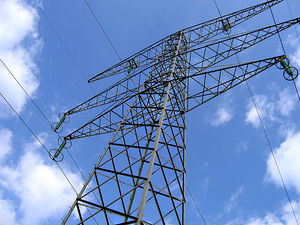Electrical conductivityFigure 1. High voltage power lines are made out of aluminum, because of it's high electrical conductivity.

Electrical conductivity is a property of materials that determines how well a given material will conduct electricity. Electrical conductivity is closely related to resistivity (which is more commonly used):

where is the conductivity (in m/Ohm) and is the resistivity (in Ohm/m). To determine the resistance of a wire (which could be made of almost anything: copper, aluminum; this equation even works for wood!), use:

where is the cross-sectional area of the wire (in m2) and is its length (in m).

Electrical conductivity is closely related to electrical conductance. Electrical conductivity is a property of the material itself (like silver), while electrical conductance is a property of a particular electrical component (like a particular wire).

Electrical conductivity can be defined as how much voltage is required to get an amount of electric current to flow. This is largely determined by the number of electrons in the outermost shell; these electrons determine the ease with which mobile electrons are generated. Another factor--but a less important one--is the number of atoms per unit volume, which determines the number of electrons that will readily move in response to an electric field. Materials with high conductivity, like copper and aluminum, are called conductors. Materials with low conductivity, like rubber and glass, are called electrical insulators (or just insulators when there's no possibility of confusion with thermal insulation). Another special class of materials like silicon and germanium are in between and are called semiconductors.

Generally, most metals have high conductivity (which is another way of saying metals tend to be conductors), because the electrons in their outermost shell can move easily. Non-metals tend to have low conductivity.